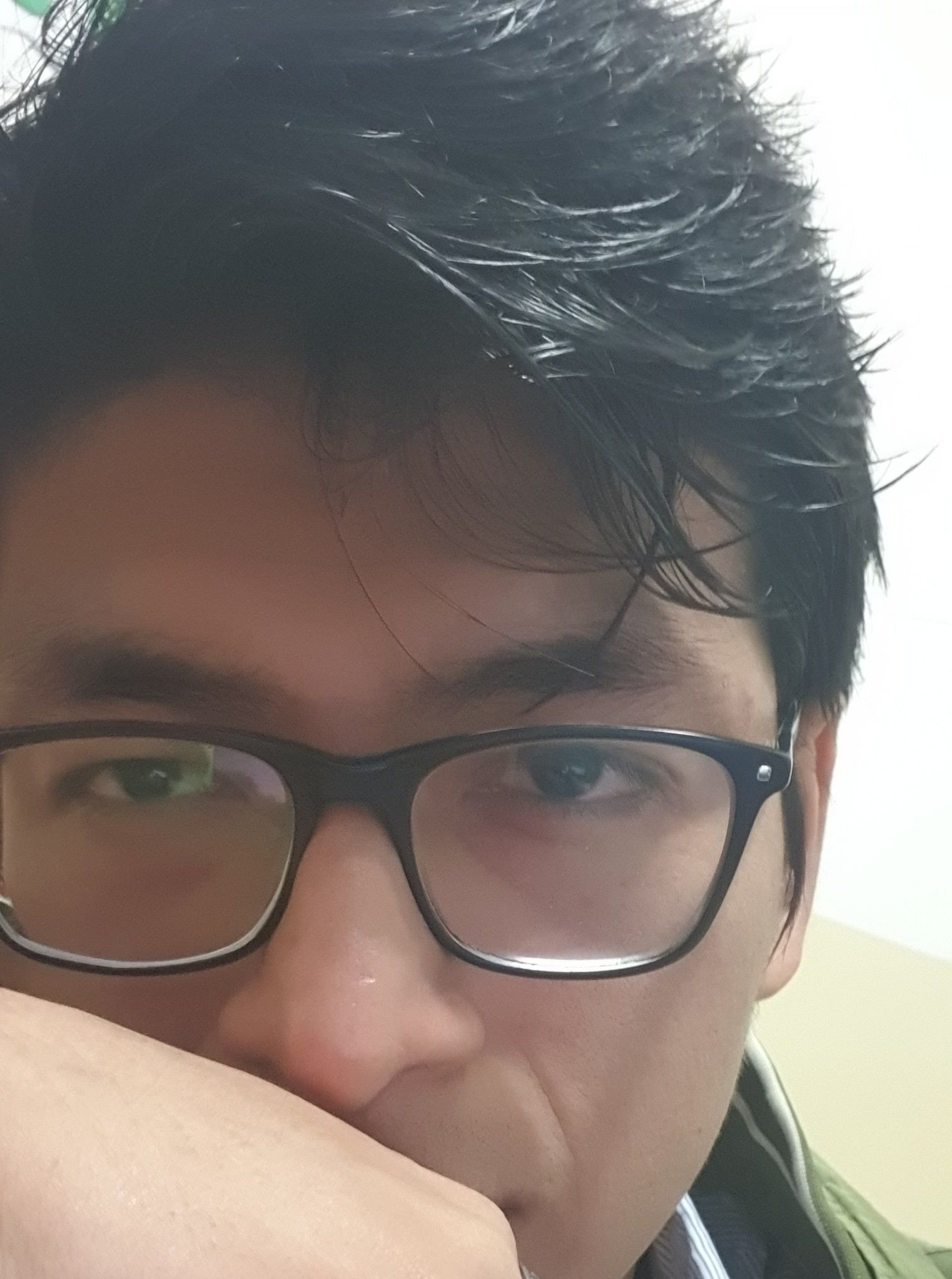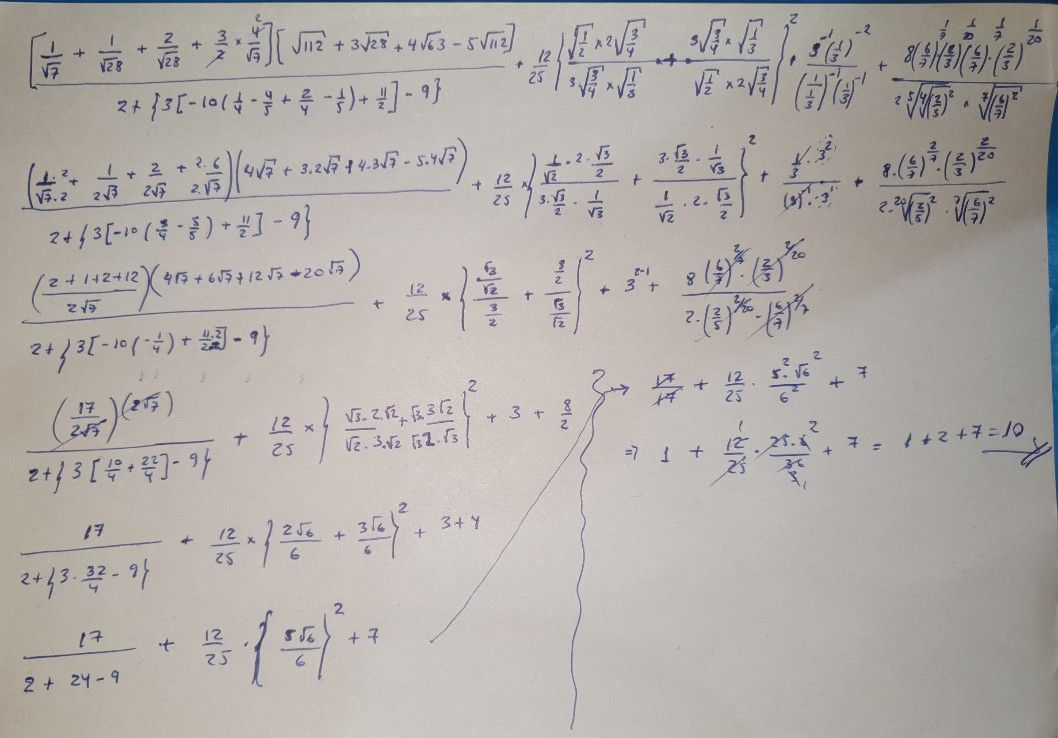Símbolos
Problema$21^{\dfrac {1} {20}}$ $617$ $21^{\dfrac {1} {20}}$ $2+ \begin{cases} 3\left(-10\left(\dfrac {1} {4}-\dfrac {4} {5}+2-\dfrac {1} {5}\right)+\dfrac {11} {2}\right)-9\right) \\ 4 \end{cases}$ $\bar{\sqrt{\dfrac {1} {2}} }$ $\dfrac {\bar{\left(\dfrac {3} {4}\right)} \times \sqrt{\dfrac {1} {3}} } {\times 2\sqrt{\left(\dfrac {3} {4}|} }\right)^{2}$ $4$ $\left(\dfrac {1} {\sqrt{7} }+-+\dfrac {2} {\sqrt{28} }+\times \sqrt{} \right)\left(\sqrt{112} +3\sqrt{26} +4\sqrt{63} -5\sqrt{112\right)}$ $\sqrt{28}$ $+\dfrac {12} {25} \begin{cases} \sqrt{1} \\ \dfrac {2^{\times 2\sqrt{\left(\dfrac {3} {4}\right)} }} {3\sqrt{|\dfrac {3} {4}|} \times \dfrac {\sqrt{1} } {3}}+ \end{cases}$ $3\sqrt{\left(\dfrac {3} {4}\right)} \times \sqrt{\dfrac {1} {3}}$ $3\longdiv{}1$ $\left(\dfrac {1} {3}$ $\left(8\right)$ $\left(6\right)^{7}$ $=$ $3|$ $\left(\dfrac {1} {3}\right)$ $\left(2\right)$ $\sqrt{\sqrt{2^{2}} }$ $\left(3\right)$ $\times 7\sqrt{\left(\dfrac {6} {7}\right)^{2}}$
Bachillerato
Otra
Búsquedas: 188
SoluciónProfesor de Qanda - Phillips